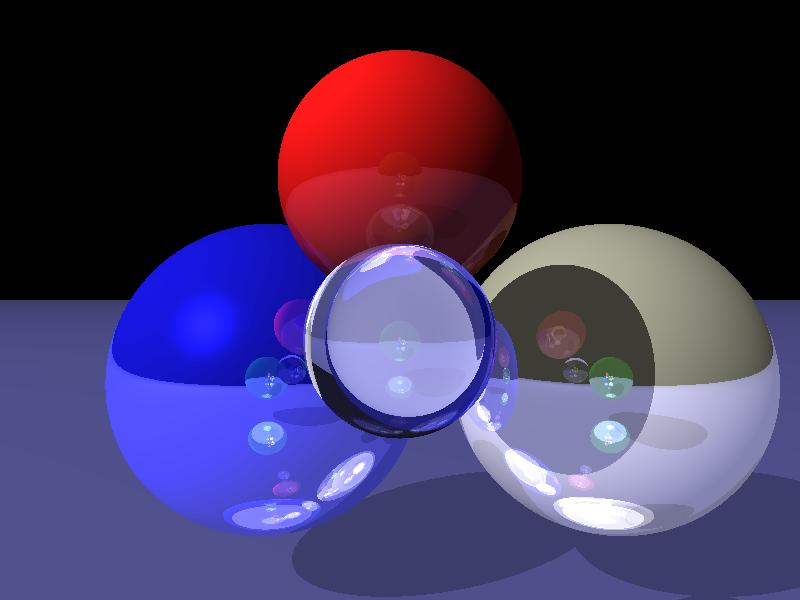# Ray Tracing

## Description

For my final project, I implemented a ray tracer in go, that can simulate light hitting an object, reflecting off the object, and refracting through the object. The ray tracer reads from a povray input file specified on the command line. I only support the lighting components (diffuse, ambient, specular, roughness), reflection, refraction, and index of refraction properties within the povray file. The ray tracer will also run the initial casts in parallel using either the number of cores on your machine, or the value of the environment variable GOMAXPROCS to determine how many threads to spawn.

## Basic Algorithm

The basic steps I followed to cast a scene are as follows:

1. Store all the objects that will be in your scene into a buffer
2. Calculate a view plane based on your camera's position, up vector, right vector, and look_at point
3. For each point in the view plane, cast a ray from your camera through the point, and write the color of the intersected object to your image
The casting also required a basic algorithm:
1. Start with an initial ray given to you, go through your buffer of saved objects, and find which object the ray will intersect first, if any
2. Test for ray intersection
1. If ray doesn't intersect any objects, return predetermined background color
2. If ray does intersect an object, save the point of intersection and which object was hit
3. Assuming there was an intersection, calculate the current pixel's color with phong shading of the intersection point using the objects diffuse, ambient, specular, and 1/roughness values
4. If the object's reflection coefficient is greater than 0, then calculate the reflected color:
1. Calculate the reflected ray starting at the intersection point, and traveling away at the same angle to the normal as the incoming ray was
2. Determine the reflected ray's color by casting your reflection ray using the same algorithm as your global casting
3. If your reflected ray hit anything, then multiply your reflected ray's color by the object's reflection coefficient, and add it to the current calculated pixel color
5. If the object's refraction coefficient is greater than 0, then calculate the refracted color:
1. Calculate the refracted ray by starting at the intersection point and calculating the ray's direction using the object's index of refraction and the equation: n1 * sin(Θ1) = n2 * sin(Θ2), where n represents the index of refraction. I assumed the non-object was air with an index of refraction of 1.0
2. Determine the refracted ray's color by casting the refracted ray using the same algorithm as the global cast
3. If your refracted ray hit anything, then multiply your refracted ray's color by the object's refraction coefficient, and add it to the current calculated pixel color
6. Finally, if the computed pixel's color had an alpha component less than 1, I needed to get the color of the object behind it, if one exists. I accomplished this by ray casting (again!) with the current object being ignored in the buffer of objects. Then multiply the returned color by 1 - (computed pixel's color).alpha and add it to the computed pixel's color

## Parallelization

In order to parallelize the ray caster, I utilized go's goroutines and channels. A goroutine can either be a new thread or process that will run the given function, depending on what the program sets up. A channel in go is essentially a pipe that allows goroutines to communicate with one another in either a synchronous or asynchronous manner. I set up the program to use goroutines as threads, and created an asynchronous channel that the main goroutine will write into and the child goroutines will read from. I decided to parallelize this program by creating a number of threads and having them read in the pixels that needed to be cast, and cast them, writing the returned color into an image data struct. This allows multiple rays to be initially cast in parallel, however any recursive casting will still be done serially.

## Sample casts

##### Reflection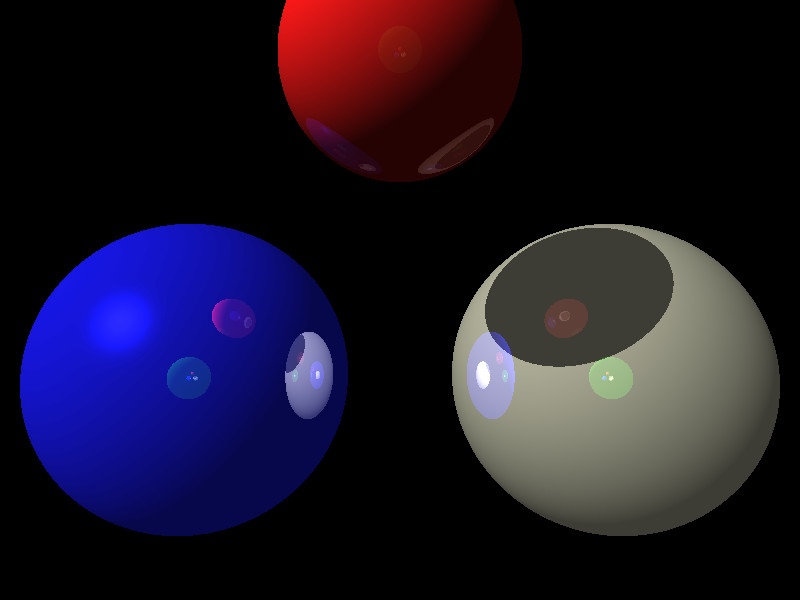##### Refraction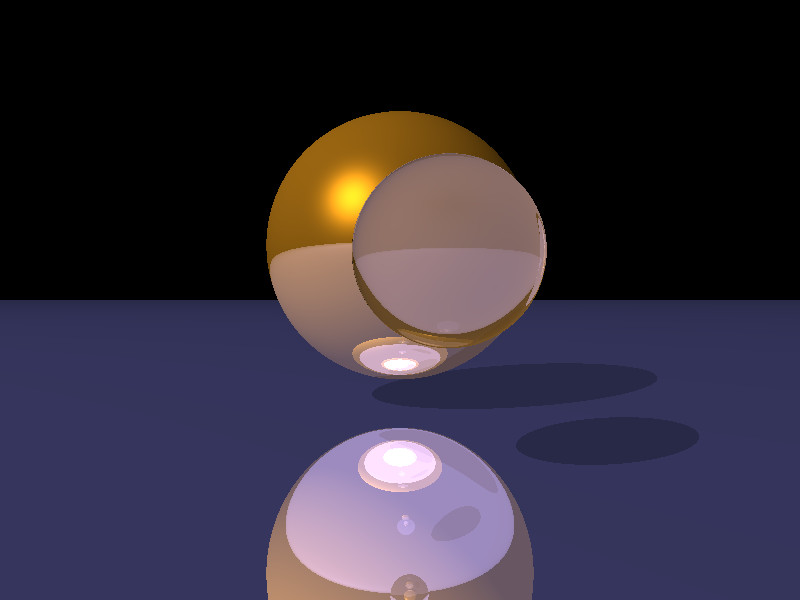##### Many Spheres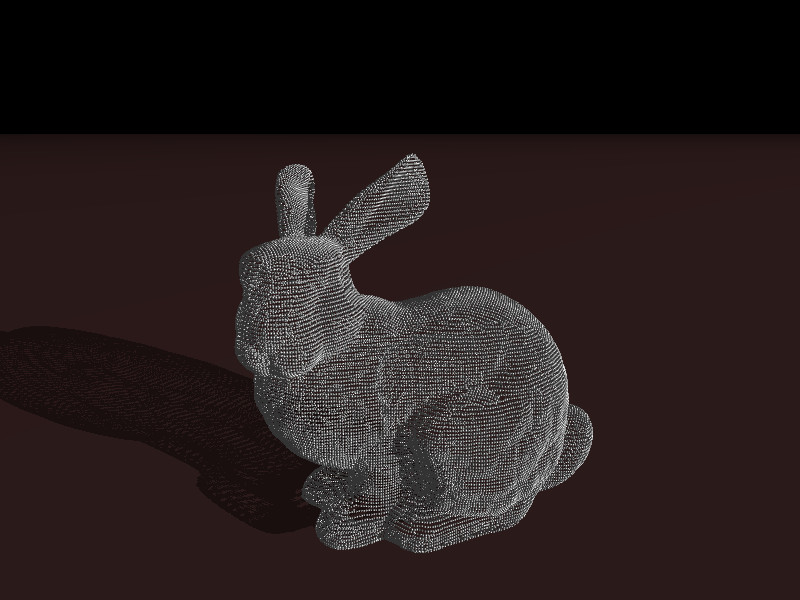##### Many Many Spheres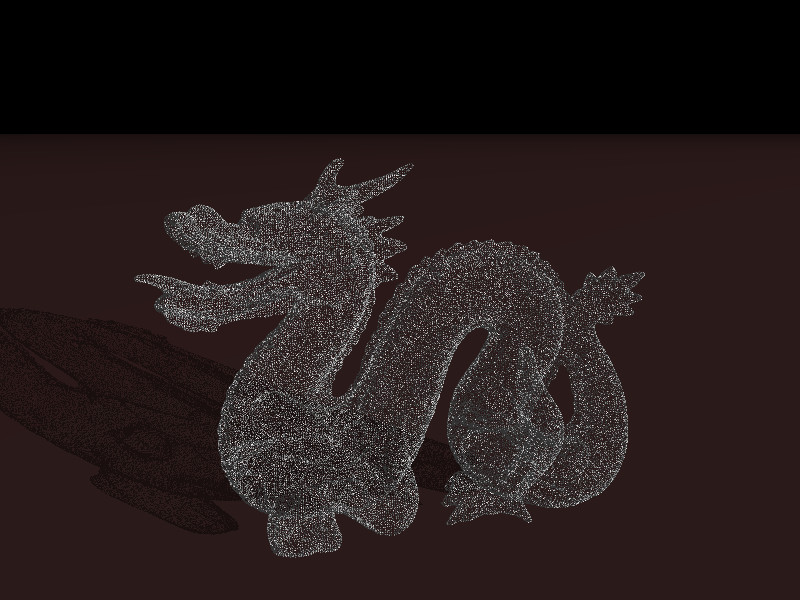##### Reflection and Refraction